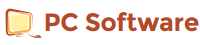### Graphulator 4.4 Saturday, September 30, 2017

Graphulator is a numerical graphing calculator for 2 or 3 variable equations. Plot both functions and non-functions. Includes data output! Now includes numerical calculus. Scientific calculator. Trigonometry. Logarithms. Absolute values. Scientific notation. Basic Calculator. Function calculator. Two variable calculator. Three variable calculator. Drag grid or use controls to move or change the scale. Drag three variable grid to rotate. Graphulator can miss non-functions 1/50 the grid scale. The user can adjust the accuracy or change the grid scale including adjusting the width and height independently. Changing the accuracy requires upgrade. Graphulator boasts being the only calculator which can perform calculus on non-functions numerically (equations which cannot be reduced to a function). It should be noted that these derivatives differ from standard calculus. Graphulator boasts being the only numerical calculator which can plot three variable non-functions. (Equations of the form f(xyz) = f(xyz)).

What's new in version 4.4
Version 4.4 now built with the HAXE programming language.